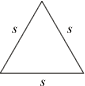# Equilateral Triangle Side Calculator

This calculator requires the use of Javascript enabled and capable browsers. This calculator is designed to give the side of an equilateral triangle, assuming the area is known. Enter the area. Then click on calculate. In a triangle, all interior angles total to 180 degrees. No two angles can total to 180 degrees or more; in an equilateral triangle, all angles are equal, or 180 degrees/3. The formula for the side is the square root(area / square root(3)/4). It is for equilateral triangles. In case you need them, here are the Trig Triangle Formula Tables, as well as the Right Triangle Angle And Side Calculator.

 Unit Number Required Data Entry Area Square Units Calculated Results Known Equilateral Side Length Units Derived Angles DegreesVersion 1.0.9

 Leave us a question or comment on FacebookSearch or Browse Our Site